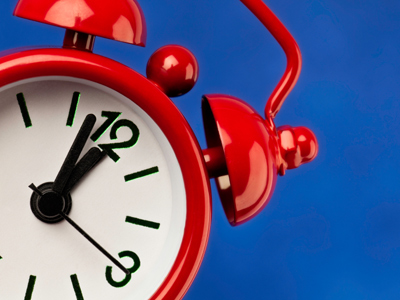When both hands are pointing to the 12, it's 12 o'clock.

# Telling the Time

This Math quiz is called 'Telling the Time' and it has been written by teachers to help you if you are studying the subject at elementary school. Playing educational quizzes is a fun way to learn if you are in the 1st or 2nd grade - aged 6 to 8.

It costs only \$12.50 per month to play this quiz and over 3,500 others that help you with your school work. You can subscribe on the page at Join Us

The first experience young children have of telling the time is usually when they learn how to say what hour it is (one o'clock, two o'clock and so on) by recognizing the longer minute hand is pointing to the 12. This knowledge of telling the time gradually extends to recognizing that when the minute hand points to the 6, it is half past the hour (half past one, half past two and so on).

Can you tell the time?

Click on the pictures for a closer look.

1.
What time does this clock show?
12 o'clock
9 o'clock
2 o'clock
10 o'clock
The minute hand is pointing to the 12 and the hour hand is pointing to the 9
2.
When it is half past, the longer minute hand points to which number?
12
6
4
8
The minute hand points straight down for half past
3.
What time does this clock show?
3 o'clock
1 o'clock
12 o'clock
4 o'clock
The hour hand shows us that it's 1 o'clock
4.
When it's 'o'clock', the longer hand is pointing to which number?
12
6
2
1
The minute hand must point to the 12 for it to be something o'clock. The shorter hand points to the hour
5.
What time does this clock show?
Half past 4
Half past 6
Half past 3
Half past 7
The minute hand is pointing straight down so it's half past. The hour hand is between the 6 and the 7
6.
What time does this clock show?
Half past 6
Half past 7
Half past 8
Half past 9
The hour hand is halfway between 7 and 8
7.
What time does this clock show?
Half past 7
Half past 9
Half past 6
Half past 8
The hour hand is half way between the 8 and the 9, so it is halfway between 8 o'clock and 9 o'clock
8.
What time does this clock show?
Half past 6
Half past 3
Half past 1
Half past 5
The minute hand is pointing to the 6 so it's half past. The hour hand is halfway between 1 and 2
9.
What time does this clock show?
5 o'clock
4 o'clock
12 o'clock
8 o'clock
The hour hand is pointing to the 8
10.
What time does this clock show?
8 o'clock
6 o'clock
1 o'clock
12 o'clock
When both hands point to the 12, it is 12 o'clock
Author:  Angela Smith